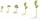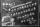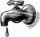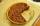Tailor 5

A tailor uses 13/4m of cloth to stitch a skirt. How many metres of cloth is needed to stitch 16 skirts?

Result

x =  52 m

Solution:Leave us a comment of this math problem and its solution (i.e. if it is still somewhat unclear...):Be the first to comment!To solve this verbal math problem are needed these knowledge from mathematics:

Need help calculate sum, simplify or multiply fractions? Try our fraction calculator. Do you want to convert length units?

Next similar math problems:

1. SeedsOf the 72 wet seeds 7/8 germinated. How many seeds germinate?
2. CakesOn the bowl were a few cakes. Jane ate one-third of them, Dana ate a quarter of those cakes that remained. a) What part (of the original number of cakes) Dana ate? b) At least how many cakes could be (initially) on thebowl?
3. Lengths of the poolMiguel swam 6 lengths of the pool. Mat swam 3 times as far as Miguel. Lionel swam 1/3 as far as Miguel. How many lengths did mat swim?
4. Scouts 44/7 of the students in a school are boys. If 3/8 of the boys are scouts, how many scouts are there in a school of 1878 students?
5. The tapFor one day flows 148 l of water out of the tap. How much water will flow out for 3/4 day?
6. Fraction to decimalWrite the fraction 3/22 as a decimal.
7. Pie IIVili ate three pieces of pie. If it pieces is 1/8 how much pie did he eat?
8. Addition of Roman numbersAdded together and write as decimal number: LXVII + MLXIV
9. One-third 2One-third of the people in a barangay petitioned the council to allow them to plant in vacant lots and another 1/5 of the people petitioned to have a regular garbage collection. What FRACTION of the barangay population made the petition?
10. Fraction and a decimalWrite as a fraction and a decimal. One and two plus three and five hundredths
11. Tallest and shortestJenn is the tallest on the team. He is 1 1/2 times as tall as the shortest girl whom is 4 1/4 feet tall. How tall is Jebb?
12. In fractionsAn ant climbs 2/5 of the pole on the first hour and climbs 1/4 of the pole on the next hour. What part of the pole does the ant climb in two hours?
13. ProductResult of the product of the numbers 1, 2, 3, 1, 2, 0 is:
14. Fractions 3Calculate 1/9 of 27:
15. PieVili ate three pieces of pie . If it piece is 1/8 how much pie did he eat?
16. ZdeněkZdeněk picked up 15 l of water from a 100-liter full-water barrel. Write a fraction of what part of Zdeněk's water he picked.
17. Write 3Write a real world problem involving the multiplication of a fraction and a whole number with a product that is between 8 and 10 then solve the problem